Bug fix: Integrals involving fractional powers

Maple has trouble with some integrals involving products of half-integer powers of linear terms. In some cases this produces an error message in Release 4:

> f1:= (2*x-3)^(-3/2)*x^(1/2);
int(f1,x);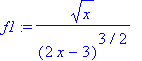Error, (in int) division by zero

In other cases the antiderivative is incorrect.

> f2:=(2*x+3)^(1/2)*x^(-5/2);
F2:=int(f2,x);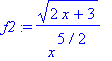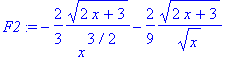> simplify(diff(F2,x) - f2);A work-around is to express the integrand as a single square root. Note that this is not quite equivalent to the original integrand (although it is when at least one of the linear terms is positive).

> f1p:= sqrt((2*x-3)^(-3)*x);
F1p:= simplify(int(f1p,x));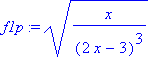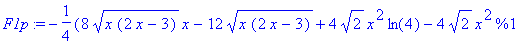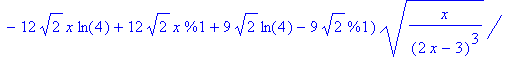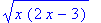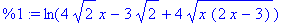> normal(diff(F1p,x)-f1p);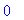> f2p:= sqrt((2*x+3)/x^5);
F2p:= simplify(int(f2p,x));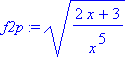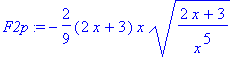> normal(diff(F2p,x)-f2p);In general it is prudent to check the correctness of integrals, either by comparing definite integrals to their floating-point approximations or by comparing the derivative of an indefinite integral to the integrand.

See also: Errors in symbolic integration , Error: division by zero , int

Maple Advisor Database R. Israel, 1998